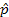## Step 3: Explore the Data

After collecting the data, Step 3 in a Statistical Investigation is to produce numerical and graphical summaries of the sample data. The goal is to discuss the overall patterns in the data. Here we don’t want to just present a bunch of "Tim" and "Bob" outcomes but a simple number and a picture or two that best represents what we learn from the data.

Taking the observational unit to be one student from class, we can define the variable as which name was put on the left, which is categorical and binary. The sample size is the number of observational units we have data for, so in this case, it equals the number of students who responded to our question in class.

### Graphical Summary, Statistic

With a binary categorical variable, we arbitrarily define one outcome to be "success" and the other to be "failure." We can then use either the number of success or the proportion of successes as the statistic.

To graph the data, we use a simple bar graph. We will use a different applet than in Lab 0 to explore these categorical data.

• In the Excel file, click on the letter A above variable name "NameOnLeft" to highlight the entire column.
• Right click and select Copy (or ctrl-C) and then in the applet below, check the Paste Data box and the Includes header box, press Clear and then right click and select Paste (or ctrl-V) to paste the data into the box. Press the Use Data button.

(a) Create a screen capture of your output (in the picture include both the summary data = (sample size n, count of successes, proportion of success, and the graph).

Also enter the values for below for later reference in the lab.

sample size (n):
# picked Tim on left (count):

After you have pasted this output in the Explore the Data section of your lab report, return to these lab instructions and press the Next button for more instructions.